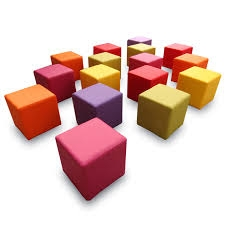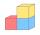# The edge of a cube

How much does the edge of a cube of 54.9 cm3 measure?

a =  3.8006 cm

### Step-by-step explanation:Did you find an error or inaccuracy? Feel free to write us. Thank you!Tips to related online calculators
Tip: Our volume units converter will help you with the conversion of volume units.

## Related math problems and questions:

• Three cubesTwo cube-shaped boxes with edges a = 70 cm; b = 90 cm must be replaced by one cube-shaped box. What will be its edge?
• Cube into cylinderIf we dip a wooden cube into a barrel with a 40cm radius, the water will rise 10 cm. What is the size of the cube edge?
• For thinkingsThe glass cube dive into the aquarium, which has a length of 25 cm, width 20 cm and height of 30 cm. Aquarium water rises by 2 cm. a) What is the volume of a cube? b) How many centimeters measure its edge?
• Cube surfce2volumeCalculate the volume of the cube if its surface is 150 cm2.
• Cube basicsHow long is the edge length of a cube with volume 15 m3?
• Cube edgeDetermine the edges of the cube when the surface is equal to 37.5 cm square.
• Cube edgesIf the edge length of the cube increases by 50%, how does the volume of this cube increase?
• The cubeThe cube has a surface of 600 cm2. What is its volume?
• Cube surface and volumeThe surface of the cube is 500 cm2, how much cm3 will be its volume?
• Volume and areaWhat is the volume of a cube which has an area of 361 cm2?
• Length of the edgeFind the length of the edge of a cube with a cm2 surface and a volume in cm3 expressed by the same number.
• Cube containersReplace the two cube-shaped containers with 0.8 dm and 0.6 dm edges with a single cube-shaped one so that it has the same volume as the two original ones together. What is the length of the edge of this cube?
• Cube diagonalsCalculate the length of the side and the diagonals of the cube with a volume of 27 cm3.
• Tetrahedral prismThe height of a regular tetrahedral prism is three times greater than the length of the base edge. Calculate the length of the base edge, if you know that the prism volume is 2187 cm3.
• CalculateCalculate the length of the wall diagonal of the cube with an edge 5 cm long.
• The cubeThe cube has a surface area of 216 dm2. Calculate: a) the content of one wall, b) edge length, c) cube volume.
• Two boxes-cubesTwo boxes cube with edges a=38 cm and b = 81 cm is to be replaced by one cube-shaped box (same overall volume). How long will be its edge?